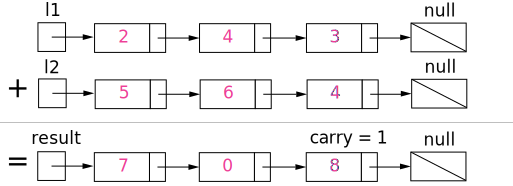Given two number represent by `LinkedList`calculate the sum of the numbers and store the result in a new LinkedList. Each node of the linked list is represented by single-digit and the head node is the most significant digit. For example:

### Example:

```Input:
First List: 3 → 6 → 5
Second List: 2 → 4 → 8

Output: (365 + 248) = 613
Resultant list: 6 → 1 → 3```

### Intuition

First, we need to reverse both the linked list. Keep track of the `carry` using a variable and simulate digits-by-digits sum starting from the head of list, which contains the least-significant digit.Visualization of the addition of two numbers: (342 + 465 = =807). Each node contains a single digit and the digits are stored in reverse order.

### Algorithm:

• Create two `LinkedList` which will represent the above two numbers.
• Reverse both the linked list.
• Add two node values (Each node is being represented as single-digit)  starting from heads of two `LinkedList`.
• If the sum is of above two node values is more than 10, then forward the carry.
• Once the addition is done then reverse the resultant `LinkedList` and return.

Just like how you would sum two numbers on a piece of paper, we begin by summing the least-significant digits, which is the head of l1 and l2. Since each digit is in the range of 0…9, summing two digits may "overflow". For example (5 + 7 =12). In this case, we set the current digit to 2 and bring over the carry = 1 to the next iteration. Carry must be either 0 or 1 because the largest possible sum of two digits (including the carry) is (9 + 9 + 1 =19).

Following is the `LinkedList `ADT  `ListNode` we are going to use it to solve the problem.

```public class ListNode {
int val;
public ListNode next;

public ListNode(int x) {
val = x;
}
}
```

Now traverse both lists. One by one pick nodes of both lists and add the values. If the sum is more than 10 then make `carry` as 1 and reduce `sum`. If one list has more elements than the other then consider remaining values of this list as 0. Following is the implementation of this approach.

```package com.tuturself.linkedlist;

public ListNode add(ListNode l1, ListNode l2) {
if (l1 == null) {
return l2;
}
if (l2 == null) {
return l1;
}
while (l1 != null && l2 != null) {
int sum = l1.val + l2.val + advance;
sum = sum % 10;
current.next = new ListNode(sum);
current = current.next;
l1 = l1.next;
l2 = l2.next;
}

if (l1 != null) {
current.next = l1;
} else {
}
} else if (l2 != null) {
current.next = l2;
} else {
}
}
// when both the lists are finished but still the carry
// is greater than 0. Example is (5+5) = 10
else if (l1 == null && l2 == null && advance > 0) {
}
}
}
```

Now let us test the program. Please use the Linked List Basic Operations article to have the basic operations of the LinkedList.

```package com.tuturself.linkedlist;

public static void main(String[] args) {

System.out.println("First List >>");
list1.print();
System.out.println("\nSecond List >>");
list2.print();
System.out.println("\nSum >>");
result.print();
}
}
```

The output of the program is:

```First List >>
1->3->5->7->9->NULL
Second List >>
2->4->6->8->1->NULL
Sum >>
3->7->1->6->1->1->NULL```

Complexity Analysis

• Time complexity : O(max(m,n)). Assume that m and n represent the length of l1 and l2 respectively, the algorithm above iterates at most max(m,n) times.

• Space complexity : O(max(m,n)). The length of the new list is at most max(m,n)+1.